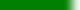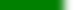# Assessable Learning Goals covered for Quiz 1 Learning GoalsDetermine what terminals are in the first set of a grammar sequence.Determine what terminals are in the follow set of a grammar symbol.Determine whether a "symbol derives to λ".Given a grammar, be able to derive a valid sentence of the grammar.How are left-associative and right-associative connected to recursive production rules?Know the difference between a leftmost derivation and a rightmost derivation.Know the notational conventions for CFGs used in the book.What are terminals, non-terminals, λ, and \$ in a CFG?What does it mean when a grammar is ambiguous?What is a sentential form?Be able to deduce associativity and precedence rules for operators in a CFG.Be able to design simple languages and grammars with nested productions and recursive rules.Given a binary operator, △, know how to write its production rule for left or right associativity.Given two binary operators △ and □ , know how to write a simple grammar to express their relative precedence.Can a BNF grammar represent different languages than a CFG?What is a parse tree, what characteristics does it have?What is a regular grammar? What two production rule patterns are associated with regular grammars?What features of a language are REs able to describe?Be able to optimize a DFA to a smaller form by reconciling non-distinguishable states.Be able to convert a DFA into a RE (in the book's notation).Cite two ways that a FA (DFA or NFA) may be represented in computer memory. Understand their tradeoffs.What are dead states and unreachable states in FAs?What is the difference(s) between a NFA and a DFA?Be able to convert an NFA to a (unoptimized) DFA.Be able to construct a RE (in the book's notation) for particular token patterns.How are the terms regular language, regular set connected to DFAs?How are the terms regular language, regular set, and regular expression interrelated?Know the (book's) notation and terminology for regular expressions.Know the meaning of RE notations: AB, A|B, A∗, A+ and Aκ (κ a constant positive integer)Know the meaning of λ, single characters s ∈ Σ, and T ⊆ Σ as they relate to the book's RE notation.What are the three basic operations in REs from which all other operations may be derived.Be able to convert a RE (in the book's notation) to an equivalent NFA.